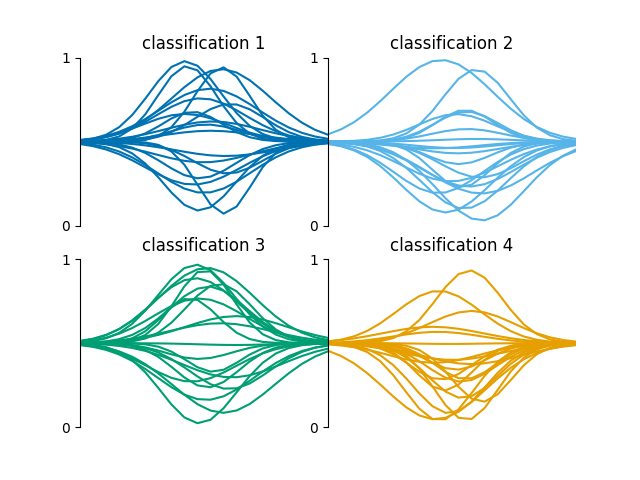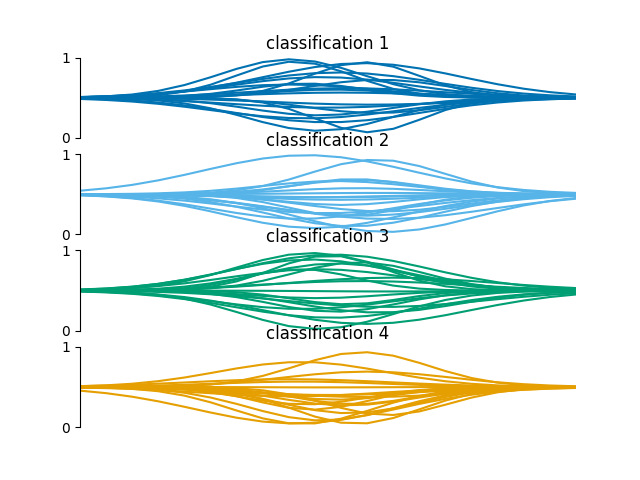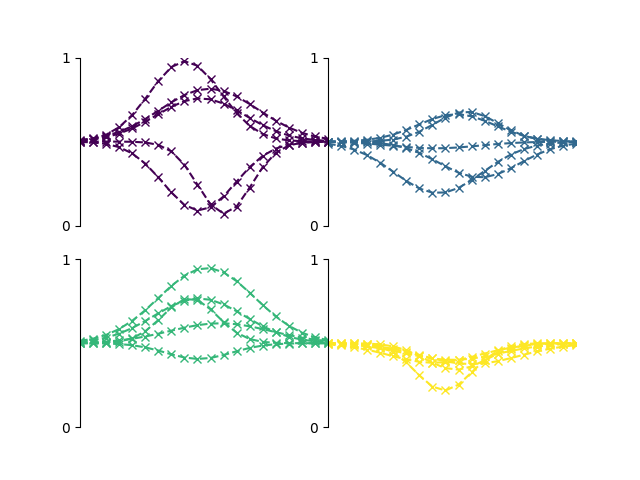# Plot a grid of spectra grouped by classification

This is an example showing how to produce a grid of line plots of an array of spectra labelled with a classification.

First we shall create a random array of spectra each labelled with a random classifications. Usually you would provide your own set of hand labelled spectra taken from spectral imaging observations of the Sun.

```from mcalf.tests.visualisation.test_classifications import spectra as s

n = 200  # 200 spectra
w = 20  # 20 wavelength points for each spectrum
low, high = 1, 5  # Possible classifications [1, 2, 3, 4]

# 2D array of spectra (n, w), 1D array of labels (n,)
spectra, labels = s(n, w, low, high)
```

Next, we shall import `mcalf.visualisation.plot_classifications()`.

```from mcalf.visualisation import plot_classifications
```

We can now plot a simple grid of the spectra grouped by their classification. By default, a maximum of 20 spectra are plotted for each classification. The first 20 spectra are selected.

```plot_classifications(spectra, labels)
```Out:

```GridSpec(2, 2)
```

A specific number of rows or columns can be requested,

```plot_classifications(spectra, labels, ncols=1)
```Out:

```GridSpec(4, 1)
```

The plot settings can be adjusted,

```plot_classifications(spectra, labels, show_labels=False, nlines=5,
style='viridis', plot_settings={'ls': '--', 'marker': 'x'})
```Out:

```GridSpec(2, 2)
```

By default, the y-axis goes from 0 to 1. This is because labelled training data will typically be rescaled between 0 and 1. However, if a particular classification has spectra that are not within 0 and 1, the y-axis limits are determined by matplotlib.

```spectra[labels == 2] += 0.5
plot_classifications(spectra, labels)
```Out:

```GridSpec(2, 2)
```

Total running time of the script: ( 0 minutes 0.654 seconds)

Gallery generated by Sphinx-Gallery